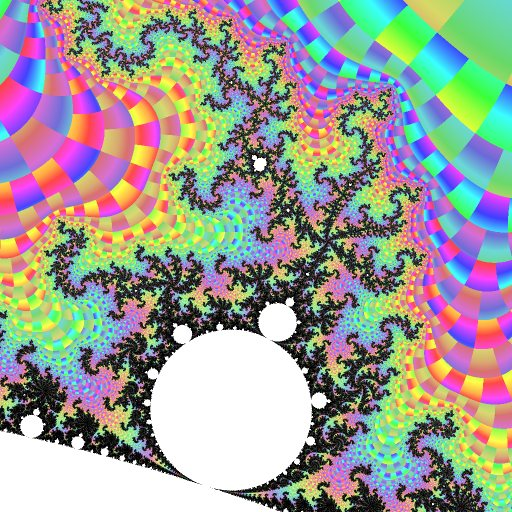# Coordinates Used as Names

Robert P. Munafo, 2008 Feb 1.

Coordinates can be used to name views, or to name features.

"View" is intended to mean an image, usually rectangular, consisting of a bunch of pixels. "Feature" is intended to mean a particular filament, or a particular mu-atom, or a particular branch point, etc.

If you are going to use coordinates to specify views of the Mandelbrot Set, it is recommended that you use the following method (or something equivalent):

1. Specify the coordinates of the point at the exact center of the image. Give enough digits so that the error is less than 1% of the size of the image.

2. Specify the size of the image (usually width and height of a rectangle). Again, enough digits must be given so that the error is less than 1%. The size is a real number giving the width or height on the complex plane — not an integer specifying the number of pixels.

Using coordinates to specify features is a more tricky process. Many features are single points, and must (in theory) be specified with precise coordinates that have an infinite number of digits. This is pretty much impossible.

For practical purposes, the best way to name a feature with coordinates is to first name a view (as above), and then give the coordinates of the feature using the same amount of accuracy. The view serves to establish a context. For example:-0.9189 + 0.2646 i @ 0.0625 ^`.C)^, _m_m_@,._c :a;\C\,_*e''^").d), ._r '@^*^*` ^^aCT-.'b, _,Y'*m\$C_^ .___:F- ^^_.b%*-^ ]@"^~"- :_Y@^m.F" '^^bc, _, .:d@F^@)c .e d-@d^ @[,d"`^\$^Y@\,'^)@@`".a:_- _, '"*"` _v@@@b'(@(d\,_\$`^ ._]bmL ., . "eF^/*b@@dY****`, :^\$C^ ___,'"mdbae,.-,___/_amdY"YbmF_-C\c _, '^"@_d*YY@Fbd@@@@\,`F@Y@)dd@@*^_^*@d@` @._ '' ./F"`a@@)ba@b(d@*^^"@Lmb/* _.. @dr ., ___%\$@F^**"^^"*-._a@F*"._ _YFYm` %^ :@^. -b-a@@@@\$F" ^Y@d@._db@F; @`m_d@dm. '^ *%@b, '@de(@bd/^ mb_C@F@@F:e,-/Y@C` Ld@@@@%^` . a, "'**,./Fd@Y@a_-*@c .dCbdmv :ad@dr ``'"^Y\d@Y_ .dd@**`. ^Y-m,, '^^"- ___e@*"L '"7L ._..*@Lmc ^`^**"ma@m@e-d@@e'*(@@@Y@@; `'^`^`^`^^'^^'^^^^^`` Center: -0.9189 + 0.2646 i Size: 0.0625 Feature: Island at -0.9153 + 0.2780 i

Here, although the coordinates of the island as given are a bit off (and don't actually fall within the island itself), the associated view coordinates make it clear that the feature you are specifying is the large, easily visible island. (Its center is actually at about -0.9164 + 0.2774 i .)

(By the way, this is the image found on the cover of the August 1985 Scientific American.)

This page was written in the "embarrassingly readable" markup language RHTF, and was last updated on 2020 Mar 26.s.11